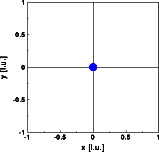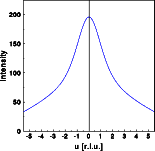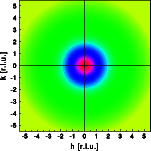Interactive Tutorial about Diffraction Basic example I: Single atomBasic examples Single atom Pair of atoms Row of atoms Goto Contents These are basic examples that familiarize students with the concept of structure simulations and Fourier transforms. The Fourier transforms of single atoms and small groups of atoms are calculated in one and two dimensions. You can either work through the examples first and then move on to the interactive simulation, or you can try running the simulations first and then verify some of your findings using the examples given. In this first example we calculate the Fourier transform of a single silicon atom. The scattering factors for X-rays are used. The atom is shown schematically as a disk. Later examples show the actual electron density distribution. The pictures below show the single atom and the corresponding 1D and 2D Fourier transform. Remember that you can get a full screen version of the pictures by clicking on them.The middle image shows a one dimensional cut through the center of reciprocal space. You can see that the intensity drops off continuously with increasing scattering vector. This is caused by the finite size of the electron density distribution at the atom. The right image shows a 2-dimensional section through reciprocal space. As depicted by the read and purple colors the intensity is highest at the origin of reciprocal space. The Fourier transform of a single atom has spherical symmetry corresponding to the spherical symmetry of the electron density distribution. © Th. Proffen and R.B. Neder, 2003